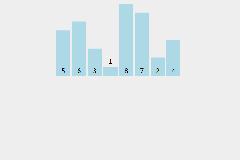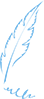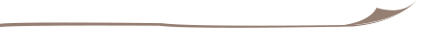# 程序猿修仙之路--算法之插入排序

12

3

1

2

3

411

2

3

C# 版本

static void Main(string[] args)

{

List<int> data = new List<int>() ;

for (int i = 0; i < 10; i++)

{

data.Add(new Random(Guid.NewGuid().GetHashCode()).Next(1, 100));

}

//打印原始数组值

Console.WriteLine($"原始数据： {string.Join(",", data)}"); int n = data.Count; //此处可以直接从第二个元素开始 for (int i = 1; i < n; i++) { //查找最小的元素的索引 for (int j = i; j>0 ; j--) { if (data[j] < data[j - 1]) { //异或法 交换两个变量，不用临时变量 data[j] = data[j] ^ data[j-1]; data[j-1] = data[j] ^ data[j - 1]; data[j ] = data[j] ^ data[j - 1]; } } } //打印排序后的数组 Console.WriteLine($"排序数据： {string.Join(",", data)}");

Console.Read();

}

Go版本

heheheheheENDposted @ 2018-11-09 21:00 架构师修行之路 阅读(...) 评论(...) 编辑 收藏